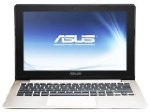## Monday, December 26, 2011

### Irregular and Regular Polygon Perimeter by Excel

Polygons that are discussed here can be regular polygon, such as: triangles, rectangles, hexagons, etc., as well as irregular polygons. This calculation can be used to measure the length of the fence of a land lot. Circumference or perimeter of a polygon is the sum of all sides. This article is related to article about the calculation of polygon area.

The figure below shows a caculation of area and perimeter for an irregular polygon shape with 19 vertices. Polygon form like this can be a piece of land lot.

As shown below, it is a polygon with a, b, c, d sides. The formula used is:

Perimeter = a + b + c + d
Where the length of each side = ((x1-x2)2 + (y1-y2)2) (1 / 2)

Draw polygon which is being measured on to cartesian coordinates, then marked each vertex with the coordinate system (x, y). Make sure the value of x and y ​​are always positive, because negative value ​​can be confusing.

Units of measurement can be anything, eg: cm, meters, miles, inches, feet, mile, etc..

To measure perimeter of a ​​land lot, firts create a drawing or map of land lot to be measured. Can use a simple yet accurate method as described on the article 'Drawing Simple Scale Map by Triangle Method'. Find the coordinates (x, y) for each vertex of land lot.

Open the attached Excel document, enter the coordinates x in the column x, and enter coordinates y in column y. Look at the chart on the right, the chart must form a desired polygon, ensure no broken line in the polygon. Chart size can be adjusted for a better fit.

Input only in the yellow cells, always start input from the top, no empty line in the middle. Should be at least one vertex touches the x axis, and one vertex touches the y axis. The first vertex is the one closest to 0 (0,0). Register vertices in sequence with clockwise direction.

Calculation for land lot area and perimeter by GPS coordinates, click here.Are you looking for a high quality laptop or notebook? Various laptop brands like Apple Macbook, Samsung Chromebook, Dell Inspiron, ASUS Vivobook, Lenovo IdeaPad, HP Pavilion and much more, can be checked in "Best Seller Laptops".

#### Post a Comment

Your positive comment will be highly appreciated to improve this site